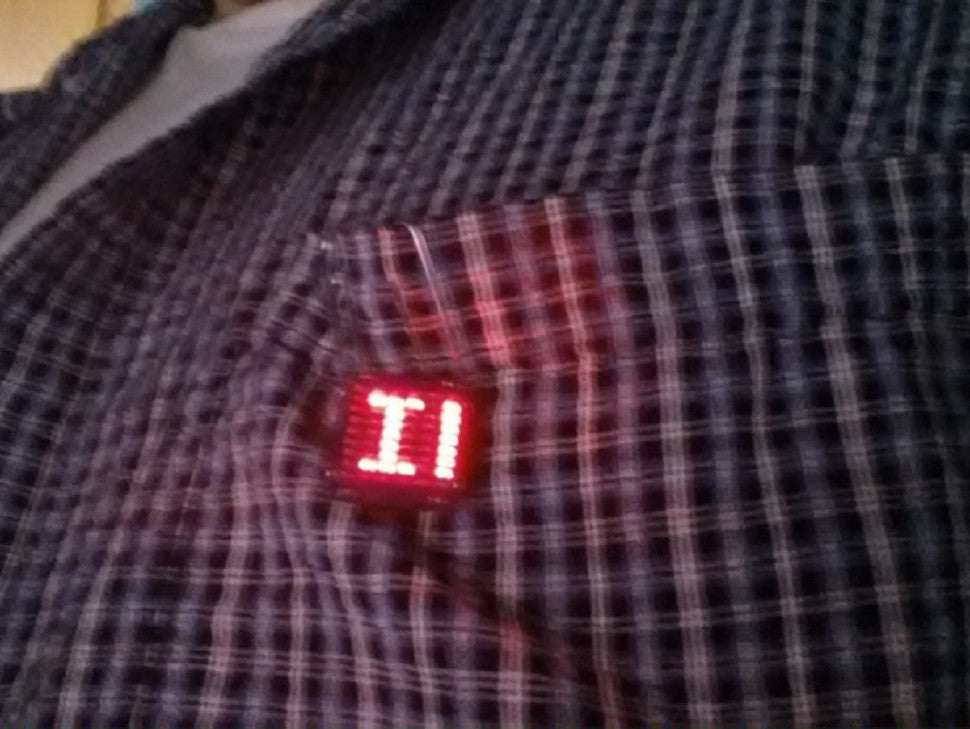# Using the Matrix LED TinyShield TutorialThe is a 6 x 9 grid of LEDs (54 total LEDs) that are individually addressable, which lets you scroll text, draw images and all sorts of other things. This TinyShield is compatible with the popular Arduino LoL Shield Library (Lots of LEDs Shield) created by Jimmie Rodgers. The LEDs are arranged using Charlieplexing, which is a technique to allow for control of multiple LEDs using fewer I/O signals. Available with Red, Green and Amber LED color options.

# Powering the System

The Matrix LED TinyShield can be run using a coin cell. The LEDs run directly off of the Vcc supply, a higher input voltage will make the LEDs brighter.# Using the Matrix LED TinyShield

The TinyShield Matrix LED shield can use the LOL Shield library from Jimmie Rodgers: with two modifications. The number of rows is different and the LED mapping is different.

Note: Unzip this library to the Arduino\libraries directory, and restart the Arduino IDE to use.

You can also modify the original LOLShield library by doing the following:

In Charlieplexing.h change the DISPLAY_ROWS definition to 9

`` #define DISPLAY_ROWS 9 // Number of rows in the display ``

In Charlieplexing.cpp change the ledMap array to match the following:

``````
const LEDPosition PROGMEM ledMap = {
L(5, 6), L(8, 6), L(6, 9), L(2, 7), L(8, 9), L(2,3),
L(6, 5), L(6, 8), L(9, 6), L(7, 2), L(9, 8), L(3,2),
L(5, 7), L(7, 6), L(6, 4), L(3, 7), L(8, 4), L(3,4),
L(7, 5), L(6, 7), L(4, 6), L(7, 3), L(4, 8), L(4,3),
L(5, 8), L(2, 5), L(6, 3), L(4, 7), L(8, 3), L(2,9),
L(8, 5), L(5, 2), L(3, 6), L(7, 4), L(3, 8), L(9,2),
L(5, 9), L(3, 5), L(6, 2), L(9, 7), L(8, 2), L(3,9),
L(9, 5), L(5, 3), L(2, 6), L(7, 9), L(2, 8), L(9,3),
L(5, 4), L(4, 5), L(7, 8), L(8, 7), L(9, 4), L(4,9)
}``````

# Examples: Scroll Text

1. Install the LOLShield Library to the Arduino\libraries\ directory
2. Restart the Arduino IDE
3. Go to the File… Menu, Examples… LOLShield… LolShield_FontTest
4. Change the text on the line that say static const char test[]=”HELLO WORLD! “; to be what you want it to scroll (Note: Text must be uppercase).
5. Upload the sketch to the TinyDuino with the Matrix LED TinyShield plugged in and watch it scroll!

# Examples: Name Tag

Download the following example sketch using the codebender plugin to make a TinyNameTag using the Matrix LED.